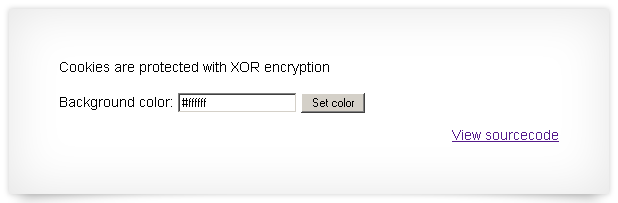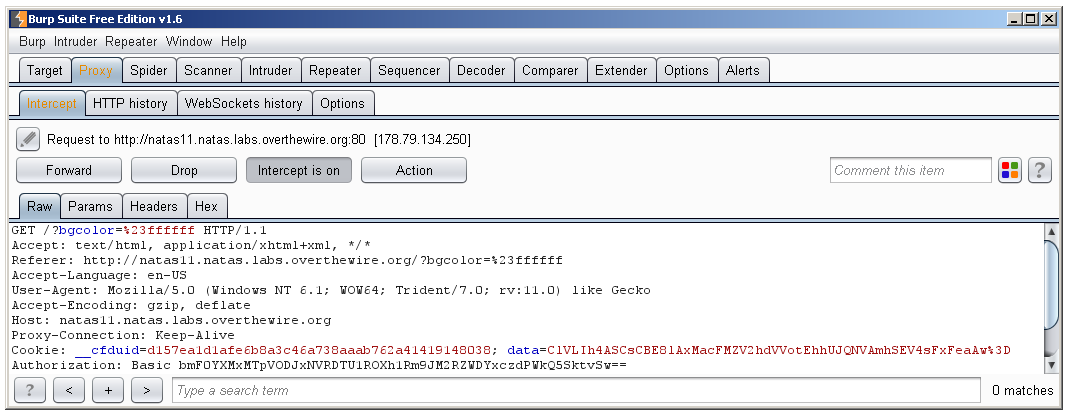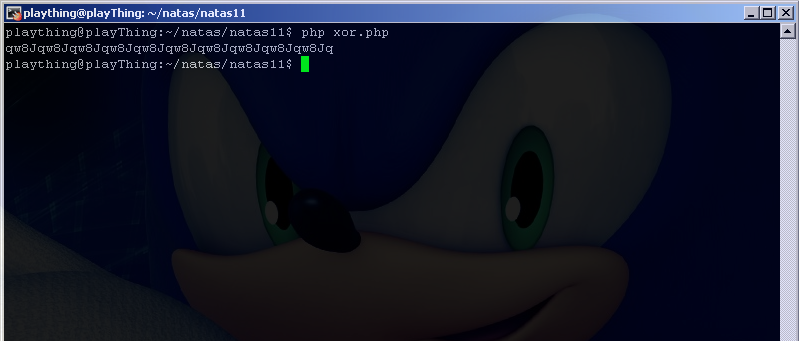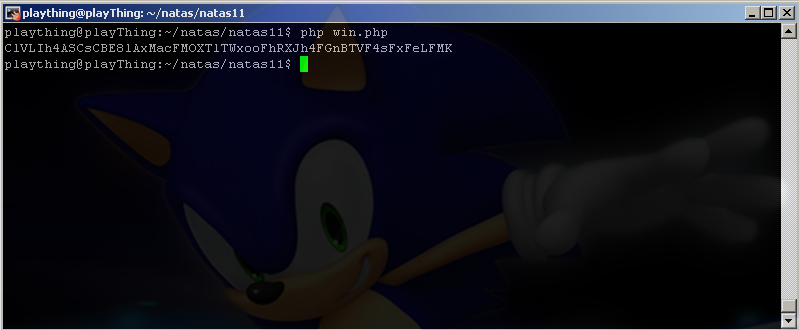# Natas Level 11This level has some meat to it so let’s jump right to the source code:

``` <html>
<!-- This stuff in the header has nothing to do with the level -->
<script src="http://natas.labs.overthewire.org/js/jquery-1.9.1.js"></script>
<script src="http://natas.labs.overthewire.org/js/jquery-ui.js"></script>
<script src=http://natas.labs.overthewire.org/js/wechall-data.js></script><script src="http://natas.labs.overthewire.org/js/wechall.js"></script>
<script>var wechallinfo = { "level": "natas11", "pass": "<censored>" };</script>
<?

function xor_encrypt(\$in) {
\$key = '<censored>';
\$outText = '';
\$text = \$in;

// Iterate through each character
for(\$i=0;\$i<strlen(\$text);\$i++) {
\$outText .= \$text[\$i] ^ \$key[\$i % strlen(\$key)];
}

return \$outText;
}

\$mydata = \$def;
if(is_array(\$tempdata) && array_key_exists("showpassword", \$tempdata) && array_key_exists("bgcolor", \$tempdata)) {
if (preg_match('/^#(?:[a-f\d]{6})\$/i', \$tempdata['bgcolor'])) {
\$mydata['bgcolor'] = \$tempdata['bgcolor'];
}
}
}
return \$mydata;
}

function saveData(\$d) {
}

if(array_key_exists("bgcolor",\$_REQUEST)) {
if (preg_match('/^#(?:[a-f\d]{6})\$/i', \$_REQUEST['bgcolor'])) {
\$data['bgcolor'] = \$_REQUEST['bgcolor'];
}
}

saveData(\$data);

?>

<h1>natas11</h1>
<div id="content">
<body style="background: <?=\$data['bgcolor']?>;">
Cookies are protected with XOR encryption<br/><br/>

<?
print "The password for natas12 is <censored><br>";
}

?>

<form>
Background color: <input name=bgcolor value="<?=\$data['bgcolor']?>">
<input type=submit value="Set color">
</form>

<div id="viewsource"><a href="index-source.html">View sourcecode</a></div>
</div>
</body>
</html>```

We’ll follow the flow of execution and take it (almost) line by line.

`\$defaultdata = array( "showpassword"=>"no", "bgcolor"=>"#ffffff");`

This creates an array called \$defaultdata with two keys “showpassword” and “bgcolor” with values set to “no” and “#ffffff” respectively.

`\$data = loadData(\$defaultdata);`

This sets the value of \$data equal to the return value of loadData(\$defaultdata).  We’ll jump inside the function to see what exactly it returns.

`global \$_COOKIE;`

According to php.net this line is needless as “[\$_COOKIE] is a ‘superglobal’, or automatic global, variable. This simply means that it is available in all scopes throughout a script. There is no need to do global \$variable; to access it within functions or methods. ”

`\$tempdata = json_decode(xor_encrypt(base64_decode(\$_COOKIE["data"])), true);`

First, the “data” key of the cookie is base_64 decoded.  What is that value anyway?  We can see it by looking at the cookie field in the HTTP header:After it’s base64 decoded it is passed as an argument to xor_encrypt, so we’ll jump inside that function.

```function xor_encrypt(\$in) {
\$key = '<censored>';
\$outText = '';
\$text = \$in;

// Iterate through each character
for(\$i=0;\$i<strlen(\$text);\$i++) {
\$outText .= \$text[\$i] ^ \$key[\$i % strlen(\$key)];
}

return \$outText;
}```

The first line of the function sets the XOR encryption key, which is our ticket to beating this level, and is censored for that reason.  Next \$outText is initialized. Then \$text is set to \$in which in this case is the base 64 decoded value of \$_COOKIE.  A for loop is set up to loop once for each character in \$text.  Inside the loop \$outText is appended by the the value resulting from \$text[\$] being XORd with \$key[\$i % strlen(\$key)] (^ is the XOR operator in php).  That might seem like a convoluted and confusing array index so I’ll take a moment to explain it.  % is the modulus operator, and it spits out the remainder after division is performed.  It is useful for creating repeating patterns of numbers and I’ll provide a brief example.  If the length of \$key is 5 and the length of text is 20, “\$i % strlen(\$key)” would look like this:

\$i = 0   and \$i % 5 = 0
\$i = 1   and \$i % 5 = 1
\$i = 2   and \$i % 5 = 2
\$i = 3   and \$i % 5 = 3
\$i = 4   and \$i % 5 = 4
\$i = 5   and \$i % 5 = 0
\$i = 6   and \$i % 5 = 1
\$i = 7   and \$i % 5 = 2
\$i = 8   and \$i % 5 = 3
\$i = 9   and \$i % 5 = 4
\$i = 10 and \$i % 5 = 0
\$i = 11 and \$i % 5 = 1
\$i = 12 and \$i % 5 = 2
\$i = 13 and \$i % 5 = 3
\$i = 14 and \$i % 5 = 4
\$i = 15 and \$i % 5 = 0
\$i = 16 and \$i % 5 = 1
\$i = 17 and \$i % 5 = 2
\$i = 18 and \$i % 5 = 3
\$i = 19 and \$i % 5 = 4

So \$key will continue iterating over \$text for each character in \$text.

Once this is done \$outText is returned.  Jumping back into loadData() \$outText is then json_decoded and \$tempdata is set.  Next 4 conditions are tested for.  First the existence of the “data” key in the \$_COOKIE field of our HTTP header.  Then we test to see if \$tempdata is an array, if it is we test whether that array contains a key called “showpassword”, and if so we test to see if there is a key called “bgcolor”.  If those conditions are all met, \$mydata[‘showpassword’] is set equal to \$tempdata[‘showpassword’], and this will be crucial in our ability to cause unintended results.  Finally we return \$mydata and \$data is set.  \$data is now looks like the following, if you left bgcolor set to #ffffff in the form:

We can safely ignore the two conditions that follow as they are concerned only with bgcolor and have nothing to do with us winning.

After that savaData(\$data) is called.  saveData() sets the “data” key of the \$_COOKIE by first json_encoding \$data, then xor_encrypting it, and finally base 64 encoding it.

Finally we have this last condition:

```if(\$data["showpassword"] == "yes") {
print "The password for natas12 is <censored><br>";
}```

So all we have to do is set our cookie to the following for the win:

Unfortunately, when we set it, it must be in their encoding scheme, which includes the XOR encryption, and we don’t have the key 🙁

We do have a way to figure out the key though, and that is because we know the clear text and cipher text values of \$data.  If we XOR the two together we should get the key.  Let’s write our own script to perform this and see what we get.

```<?php

\$encrypted = base64_decode("ClVLIh4ASCsCBE8lAxMacFMZV2hdVVotEhhUJQNVAmhSEV4sFxFeaAw=");

for (\$i = 0; \$i < strlen(\$encrypted); \$i++) {
print \$encrypted[\$i] ^ \$clear[\$i];
}
echo "\n";```

This script just goes along and XORs each plain text character with its cipher text counter part one by one and spits out the result:“qw8J” is what is repeating so that must be the key.  Let’s use another script to encode {“showpassword”:”yes”,”bgcolor”:”#ffffff”} with their scheme, we’ll then set that as our cookie in burp suite and see what happens.

```\$defaultdata = array( "showpassword"=>"yes", "bgcolor"=>"#ffffff");

function xor_encrypt(\$in) {
\$key = 'qw8J';
\$text = \$in;
\$outText = '';

// Iterate through each character
for(\$i = 0;\$i < strlen(\$text); \$i++) {
\$outText .= \$text[\$i] ^ \$key[\$i % strlen(\$key)];
}

return \$outText;
}

function saveData(\$d) {
return base64_encode(xor_encrypt(json_encode(\$d)));
}

echo saveData(\$defaultdata)."\n";```

The output:So we throw that in the cookie and: